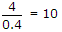# Electronics and Communication Engineering - Digital Electronics

11.

A 4 bit down counter starts counting from 1111 irrespective of modulus.

 A. True B. False

Explanation:

The starting count depends on modulus. 4 bit decade down counter starts counting from 1010.

12.

The number of counter states which an 8 bit stair step A/D converter has to pass through before conversion takes place is equal to

 A. 1 B. 8 C. 255 D. 256

Explanation:

28 = 256.

13.

74 HC series can sink upto 4 mA. The 74 LS series has IIL(max) - 0.4 mA. How many 74 LS inputs be driven by 74 HC output?

 A. 100 B. none C. 8 D. 10

Explanation:.

14.

An 8 bit transistor register has output voltage of low-high-low-high-low-high-low-high. The decimal number stored is

 A. 105 B. 95 C. 85 D. 75

Explanation:

01010101 in binary and 64 + 16 + 4 + 1 = 85 in decimal.

15.

The number of cells in a 4 variable K map is

 A. 16 B. 8 C. 4 D. 32